# 简单 Python 快乐之旅之：Python 基础语法之列表操作专题

Python 列表允许存放异构类型的元素。这和其他主流语言中的列表或者数组是不一样的。但 Python 列表将足以满足你可能需要数组的所有需求。在本文中，我们将会学习到以下内容：如何在 Python 中创建一张列表；访问列表中的元素；查找列表中元素的个数；如何添加一个新元素到列表；如何在列表中删除某项；列表元素遍历；列表排序；列表反转；以及更多 Python 列表的转化和聚合操作。

# 1. 创建一个空列表

Python 列表是一种可以存储多种不同类型元素的数据结构。

mylist = []


## 1.1. 示例：创建一个空列表

cars = []
print(type(cars))
print(len(cars))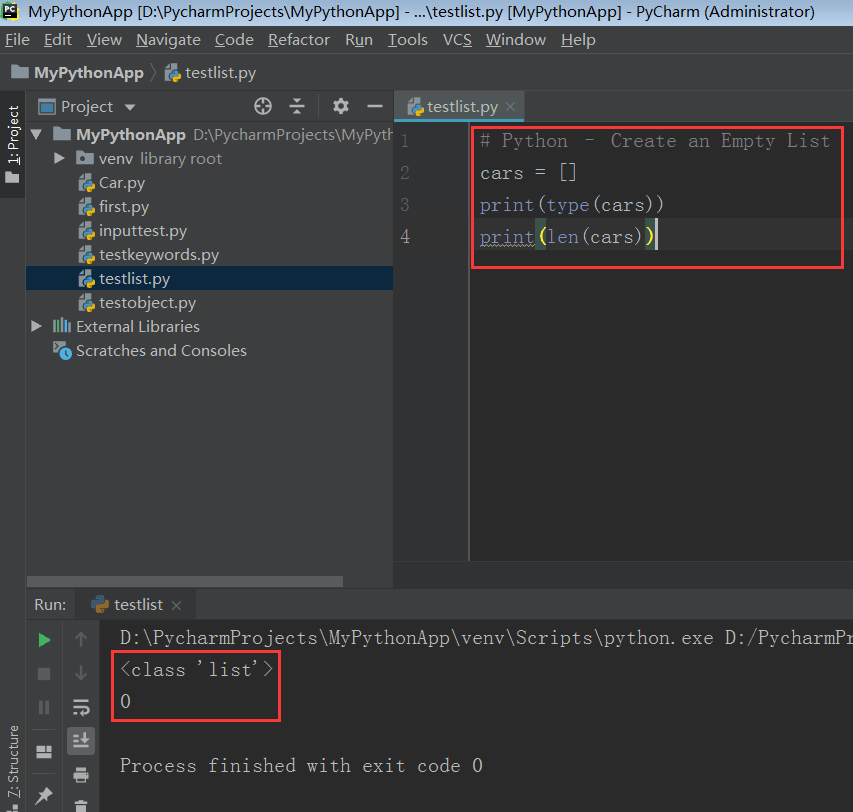# 2. 访问列表元素

mylist
mylist[2:7]


## 2.1. 示例：访问 Python 列表中的单个项

# Access a single item of Python List
a = [52, 85, 41, 'sum', 'str', 3 + 5j, 6.8]
# access 3rd item with index 2
x = a
print(x)
print(type(x))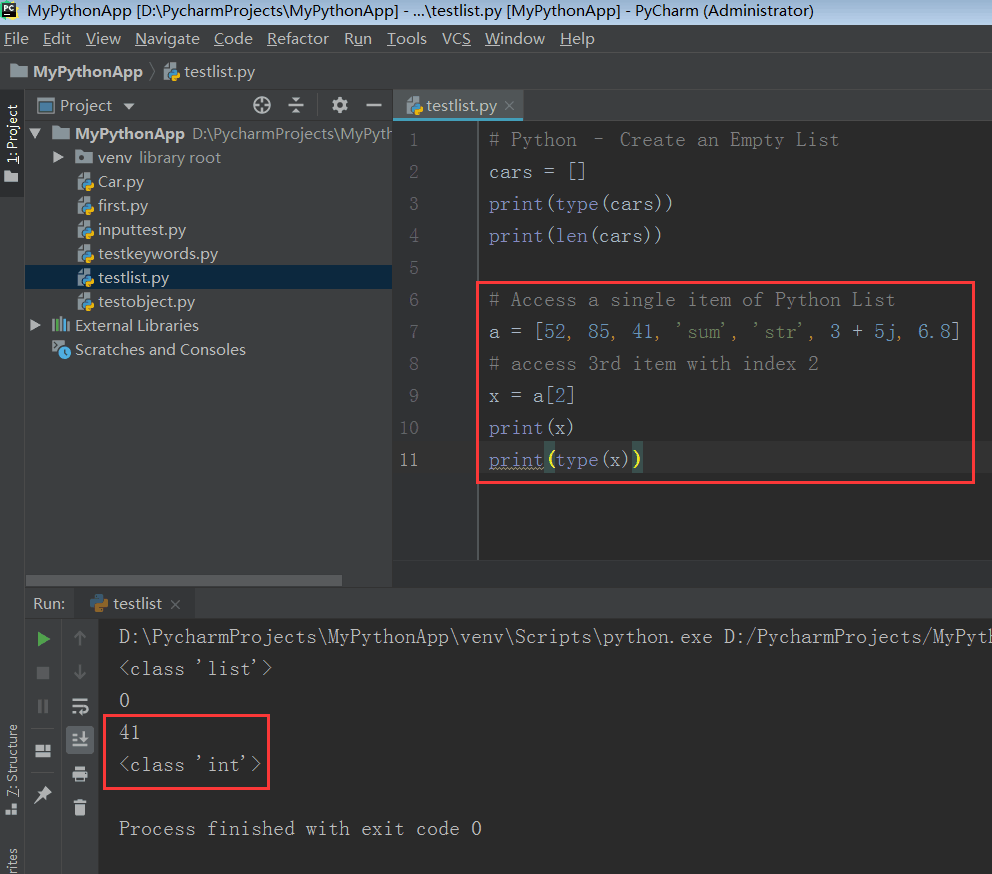## 2.2. 访问 Python 列表中的多个项

# Access a range of items in Python List
a = [52, 85, 41, 'sum', 'str', 3 + 5j, 6.8]
# access a range of items
x = a[1:4]
print(x)
print(type(x))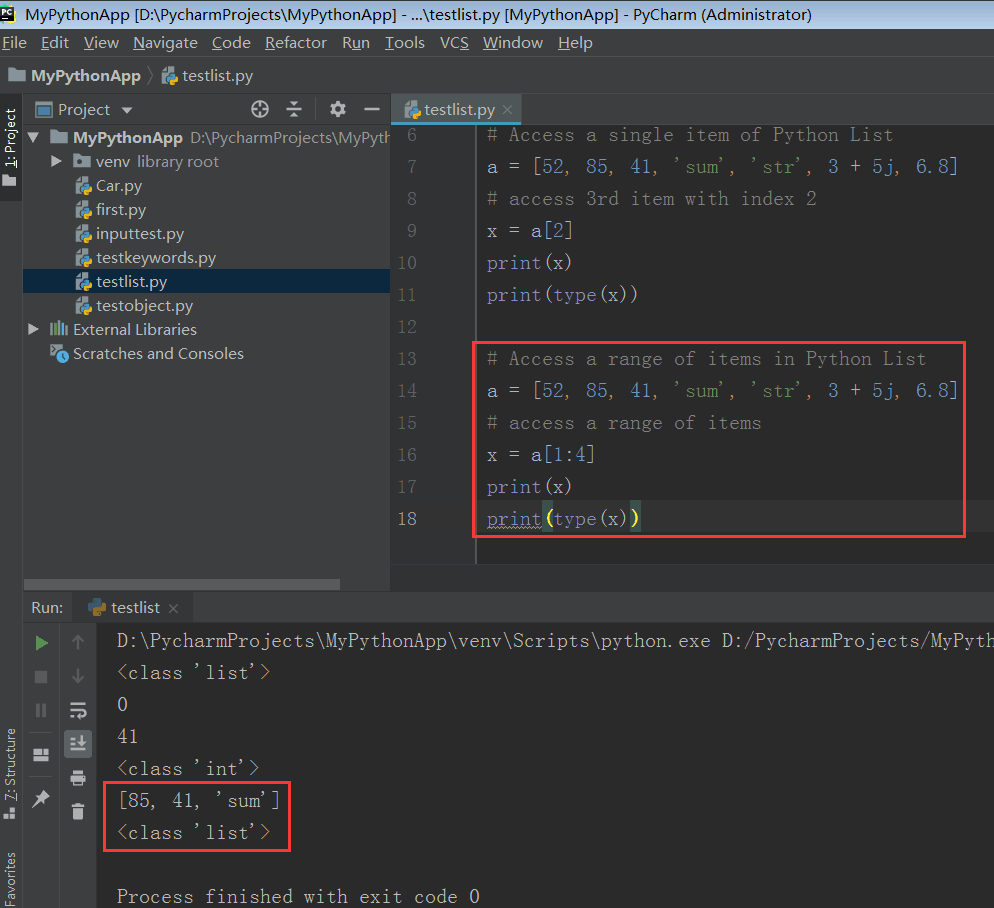# 3. 列表长度

len(listname)


# Python List Length
cars = ['Ford', 'Volvo', 'BMW', 'Tesla']
length = len(cars)
print('Length of the list is:', length)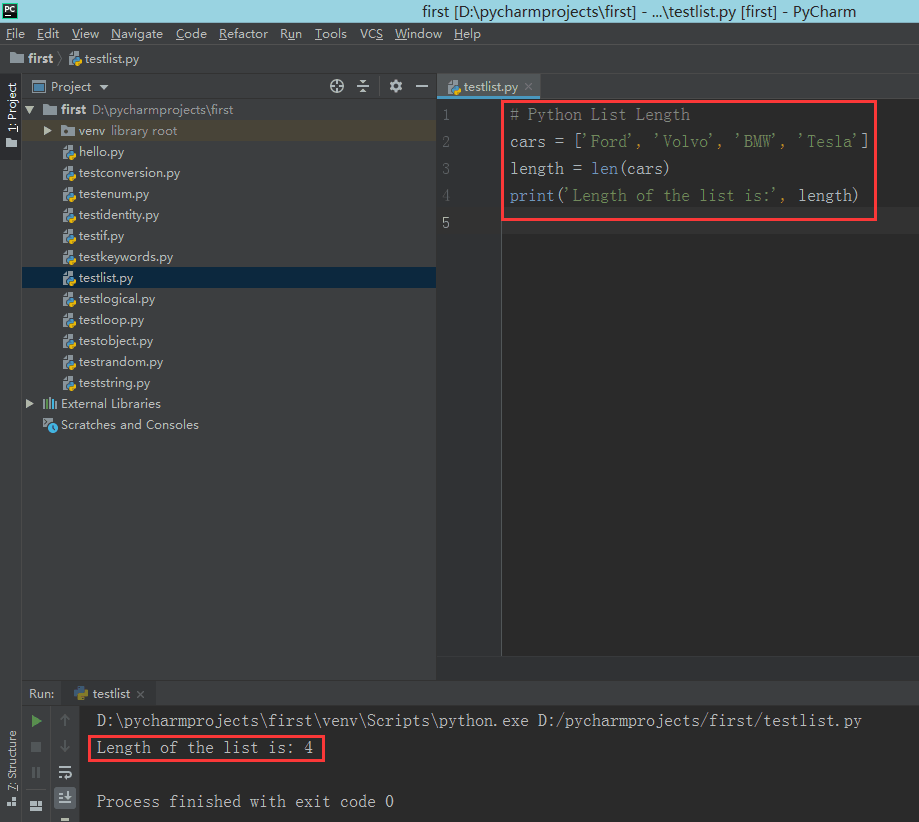# 4. 添加元素

mylist.append(new_element)


new_element 是要追加到列表 mylist 中的新元素。

## 4.1. 示例：添加新元素到列表

# Python List – Append or Add Item
cars = ['Ford', 'Volvo', 'BMW', 'Tesla']
cars.append('Audi')
print(cars)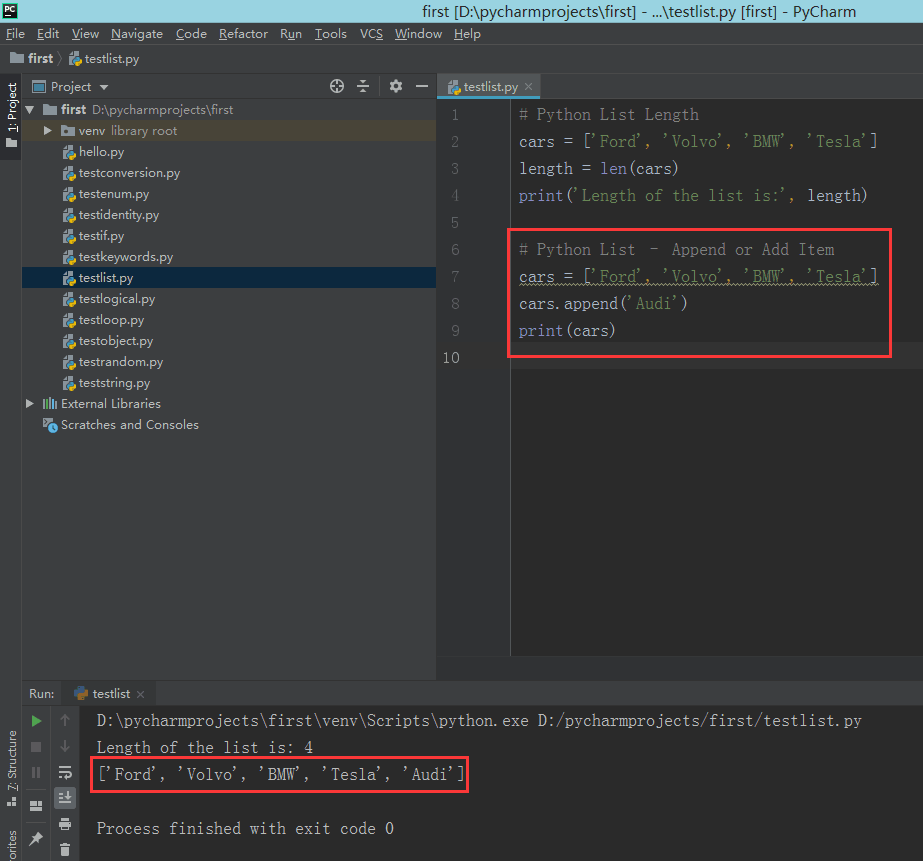# 5. 移除元素

mylist.remove(thisitem)


thisitem 是要从 mylist 中移除的那一项。
remove() 函数只会移除掉该项在列表中第一次出现的那一个，其余后续的会保留。后续我们还会学到怎么将特定值的项全部移除。

## 5.1. 移除在列表中只出现一次的元素

# Remove item that is present only once in the List
mylist = [21, 5, 8, 52, 21, 87]
item = 5
# remove the item
mylist.remove(item)
print(mylist)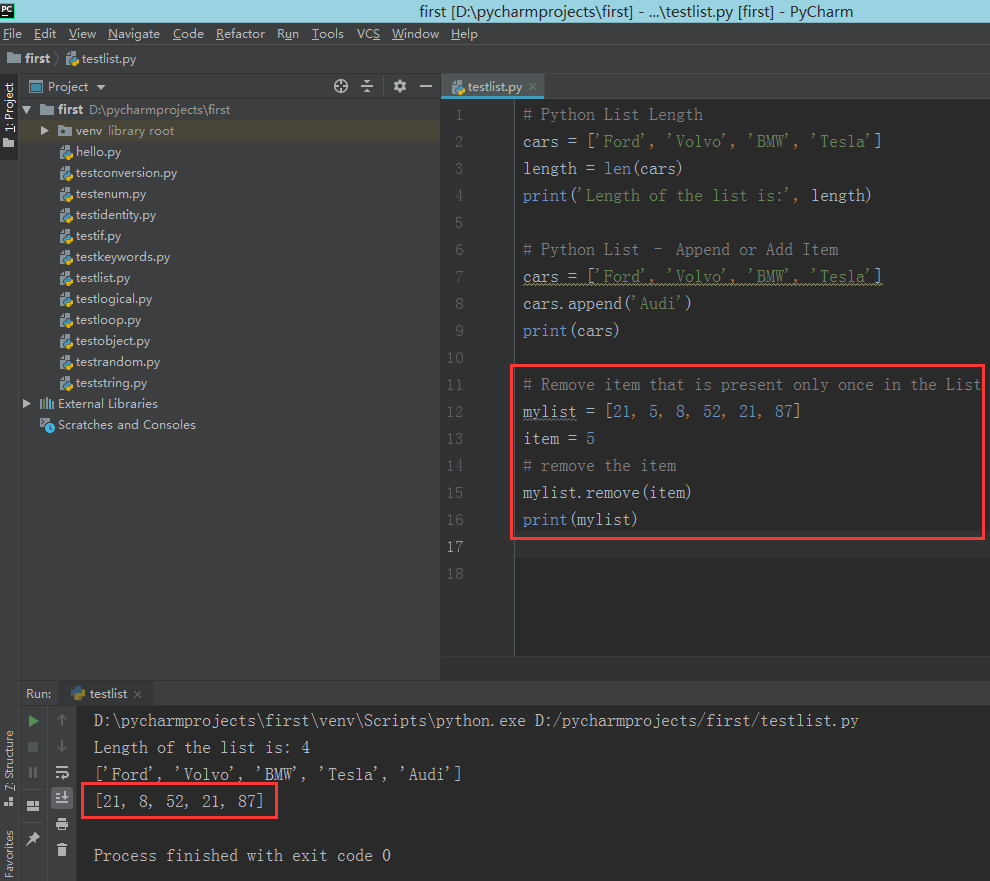## 5.2. 移除出现多次的元素

# Remove item that is present multiple times in the List
mylist = [21, 5, 8, 52, 21, 87]
item = 21
# remove the item
mylist.remove(item)
print(mylist)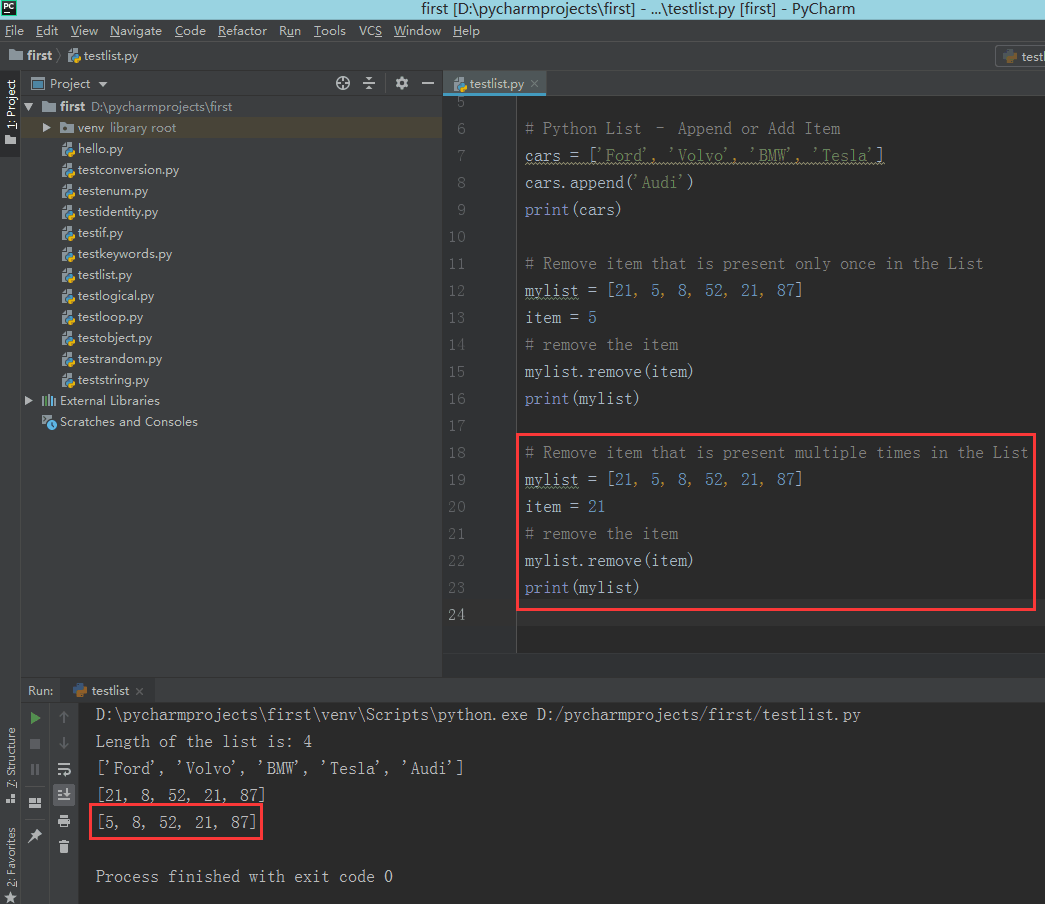## 5.3. 移除出现多次的元素的所有项

# Remove all the occurrences of an item from the List
mylist = [21, 5, 8, 52, 21, 87]
item = 21
# remove the item for all its occurrences
for r_item in mylist:
if (item == r_item):
mylist.remove(item)
print(mylist)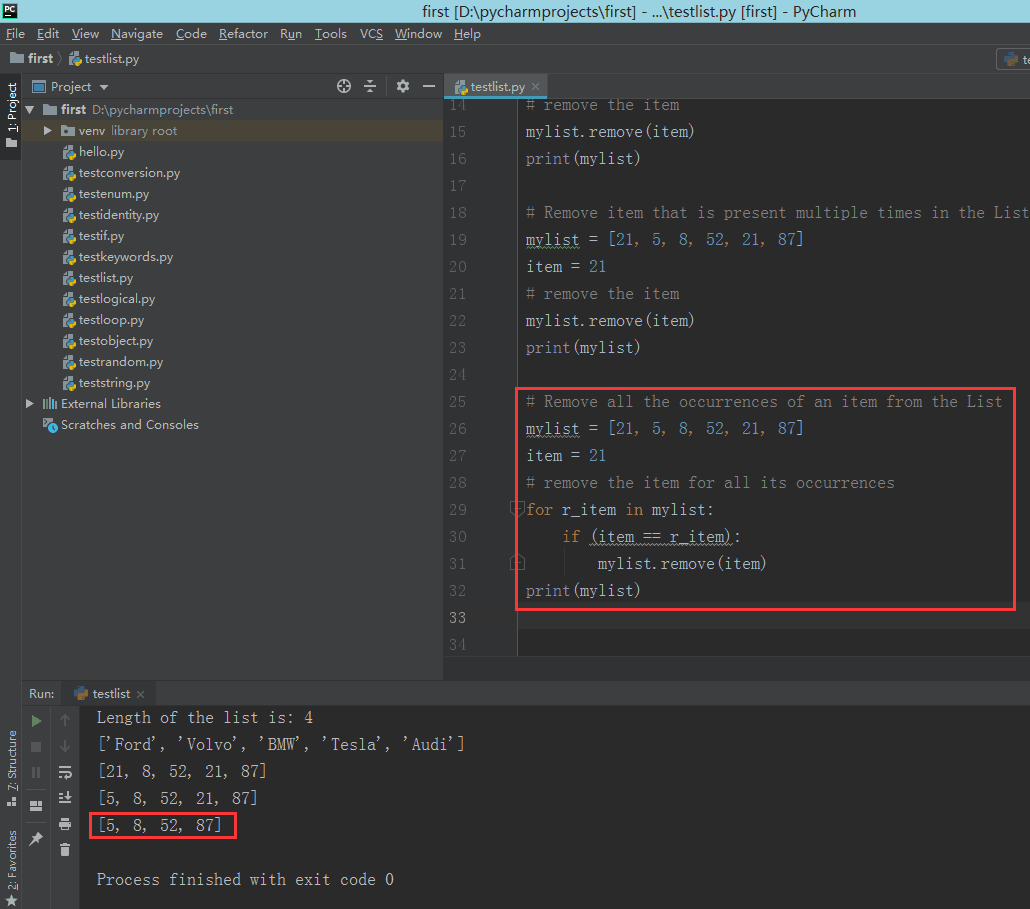# 6. 移除在列表中出现过多次的某个项

• 遍历列表并移除可以匹配到指定值的项。
• 使用 lambda 函数将不匹配到指定值的项过滤出来。
• 重复使用 remove() 方法直到指定值不存在于列表中。
推荐使用第二种方法，因为它能提供较好的性能。另外两种只是便于理解。

## 6.2. 过滤掉所有匹配项

# Remove all occurrences in List using Filter
mylist = [21, 5, 8, 52, 21, 87]
r_item = 21
# remove the item for all its occurrence
mylist = list(filter((r_item).__ne__, mylist))
print(mylist)
# Remove all occurrences in List using Filter
mylist = [21, 5, 8, 52, 21, 87]
r_item = 21
# keep the item for all its occurrence
mylist = list(filter((r_item).__eq__, mylist))
print(mylist)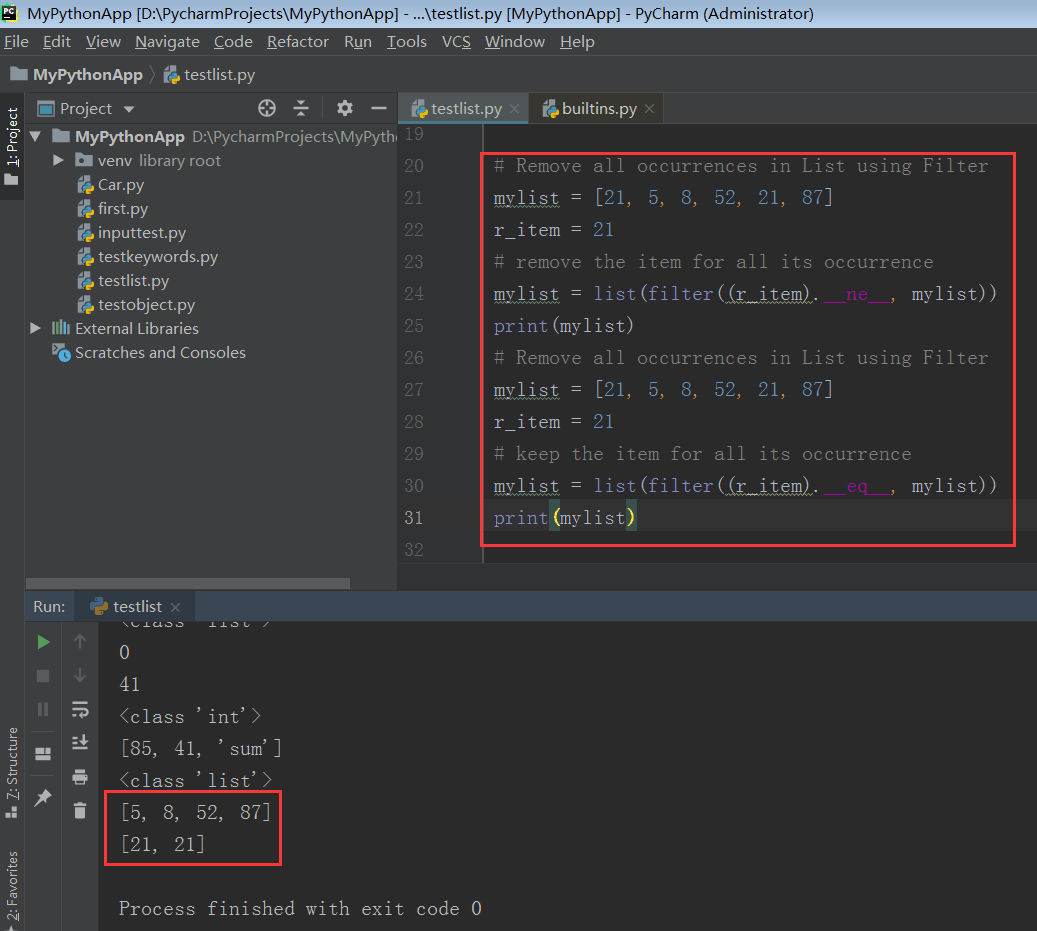## 6.3. 使用 while 循环和 remove() 方法移除

# Remove all occurrences in List using While Loop
mylist = [21, 5, 8, 52, 21, 87]
r_item = 21
# remove the item for all its occurrence
while r_item in mylist:
mylist.remove(r_item)
print(mylist)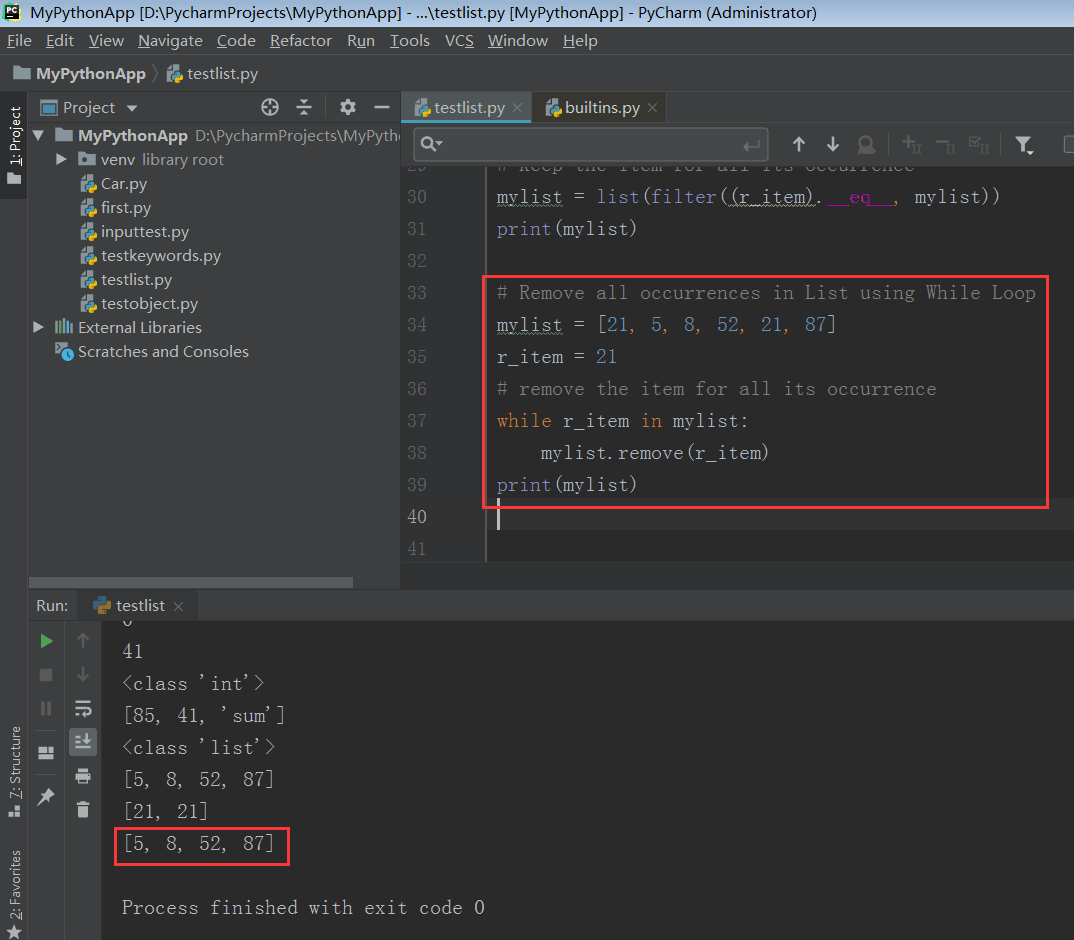# 7. 列表循环遍历

a = [52, 85, 41, 'sum', 'str', 3 + 5j, 6.8]


## 7.1. 使用 while 循环遍历

# Loop List items using While Loop
a = [52, 85, 41, 'sum', 'str', 3 + 5j, 6.8]
i = 0
while i < len(a):
print(a[i])
print(type(a[i]))
i += 1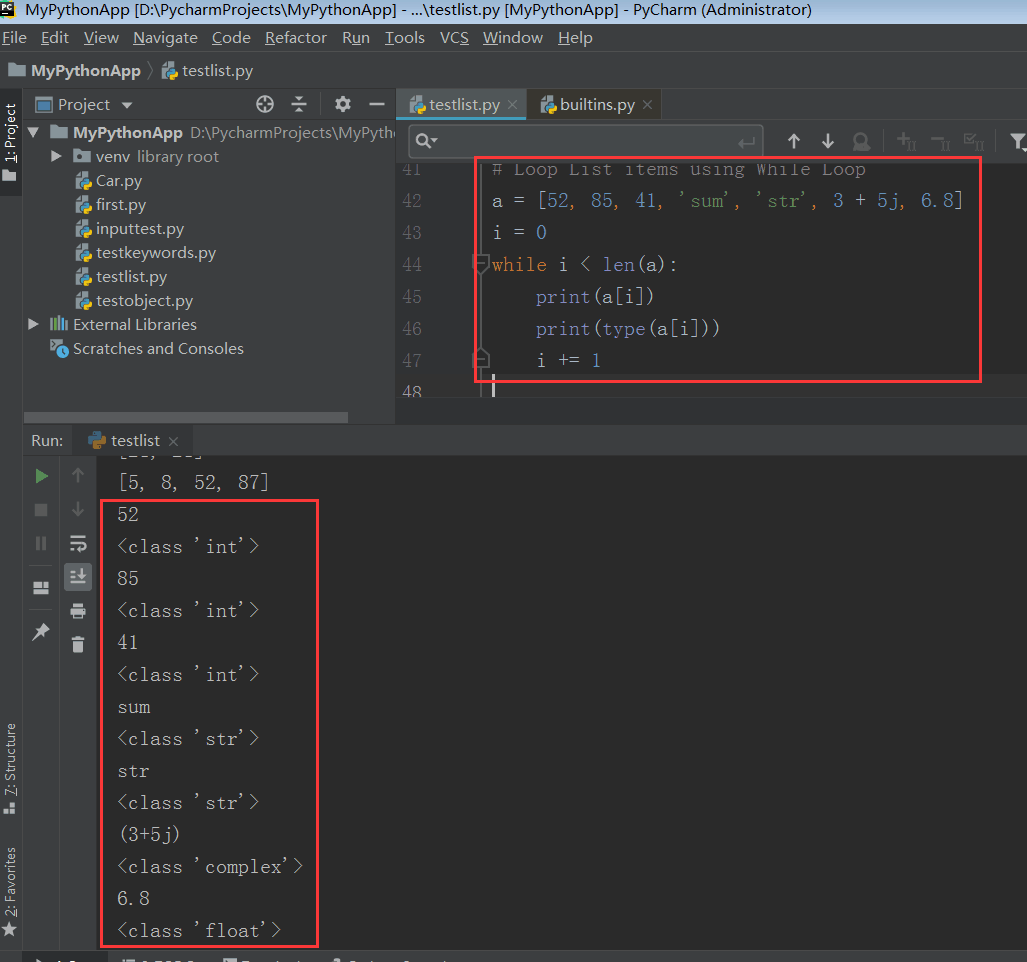## 7.2. 使用索引遍历列表

for 配合 range 函数，可以通过索引访问遍历列表。

# Loop List items using index
a = [52, 85, 41, 'sum', 'str', 3 + 5j, 6.8]
for i in range(len(a)):
print(a[i])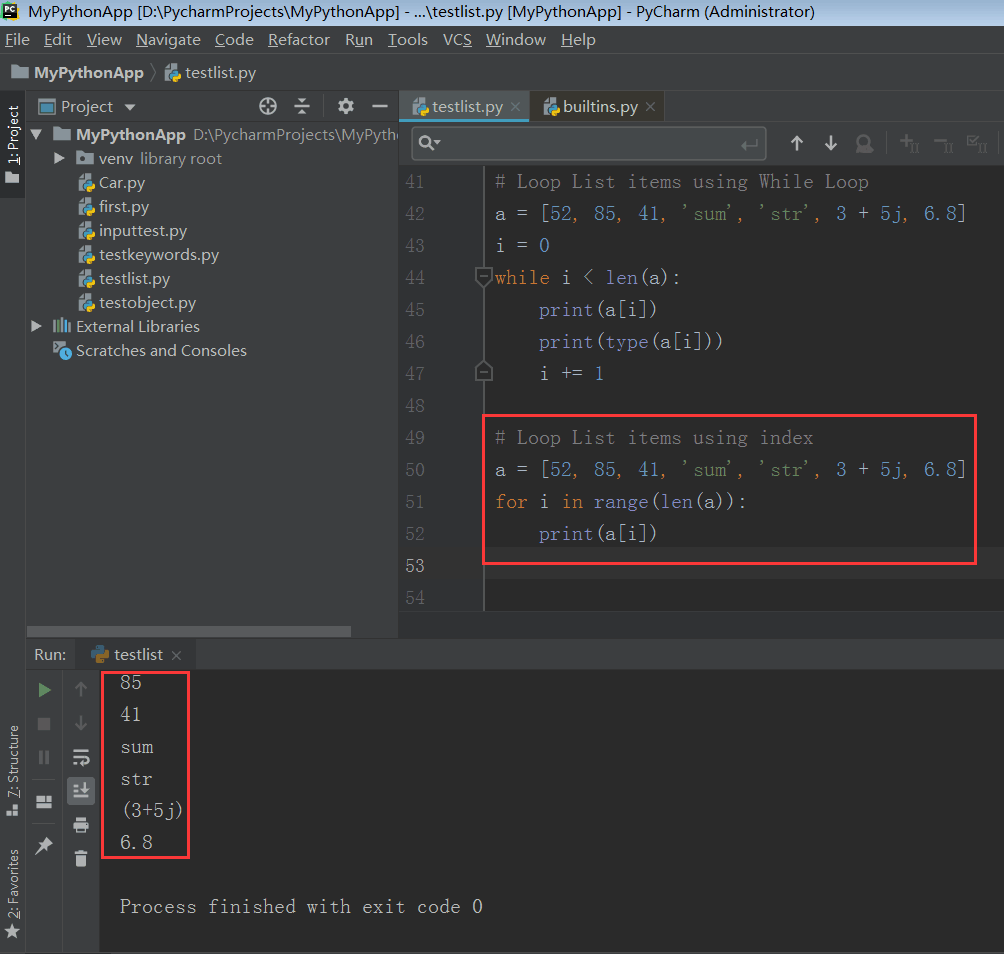## 7.3. 增强型 for 循环

# Loop List items accessing list item directly
a = [52, 85, 41, 'sum', 'str', 3 + 5j, 6.8]
for x in a:
print(x)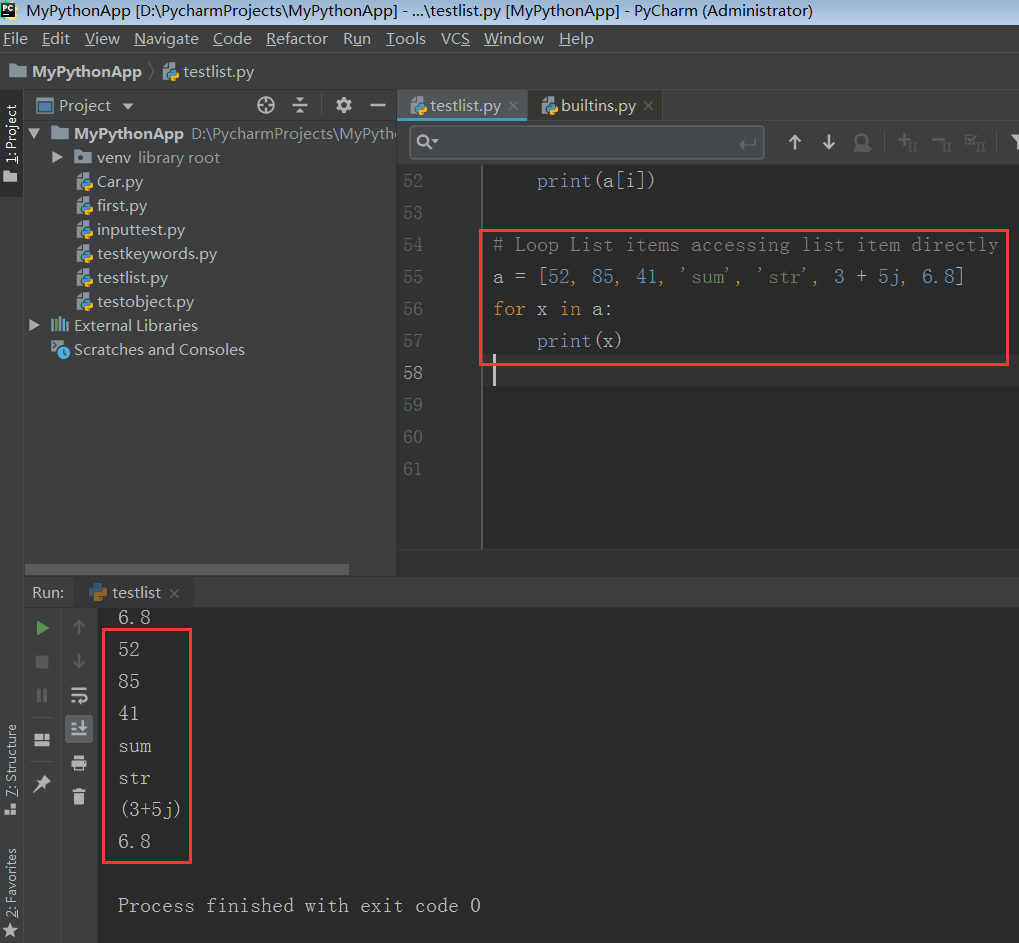## 7.4. 枚举遍历列表元素

# Loop List items using Enumerate
a = [52, 85, 41, 'sum', 'str', 3 + 5j, 6.8]
for i, x in enumerate(a):
print('element#', i, 'is:', x)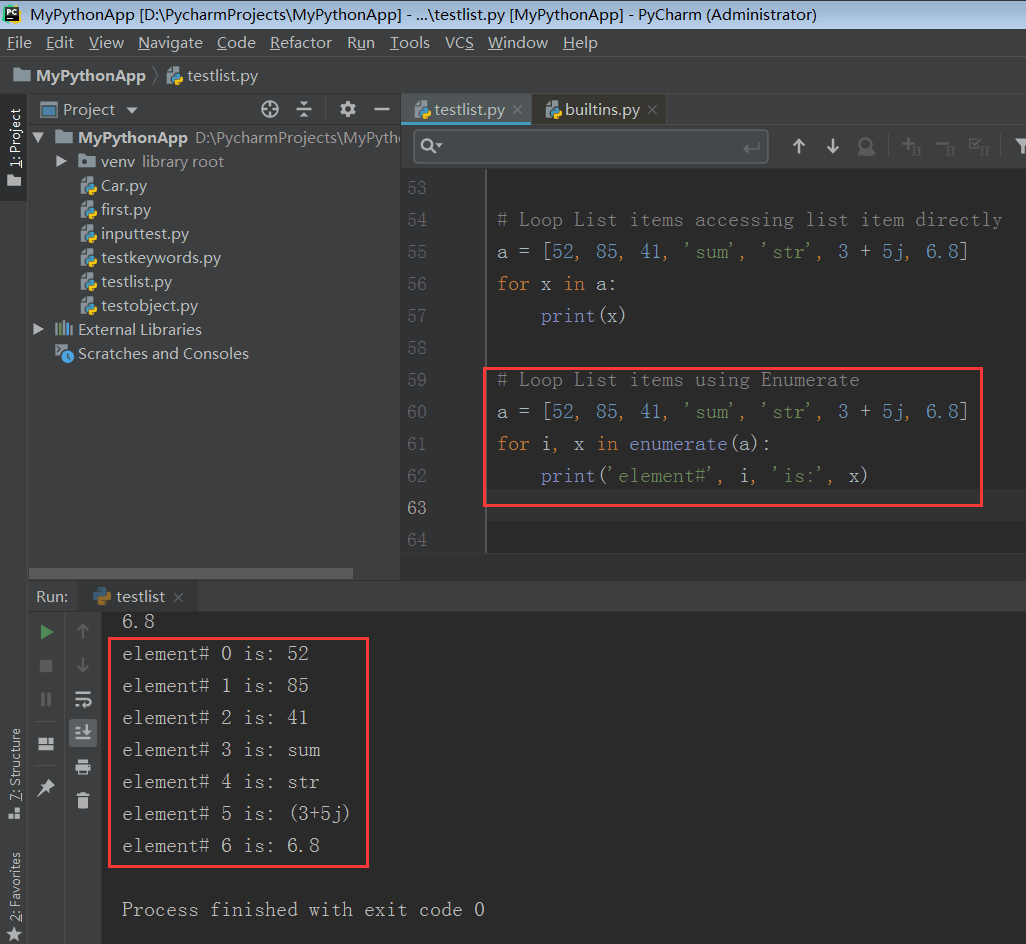# 8. 统计列表中指定值的项的个数

# Python – Count the items with a specific value in the List
mylist = [6, 52, 74, 62, 85, 62, 62, 85, 6, 92, 74]
length_74 = mylist.count(74)
length_62 = mylist.count(62)
length_92 = mylist.count(92)
length_73 = mylist.count(73)
print('74 occurred', length_74, 'times in the list')
print('62 occurred', length_62, 'times in the list')
print('92 occurred', length_92, 'times in the list')
print('73 occurred', length_73, 'times in the list')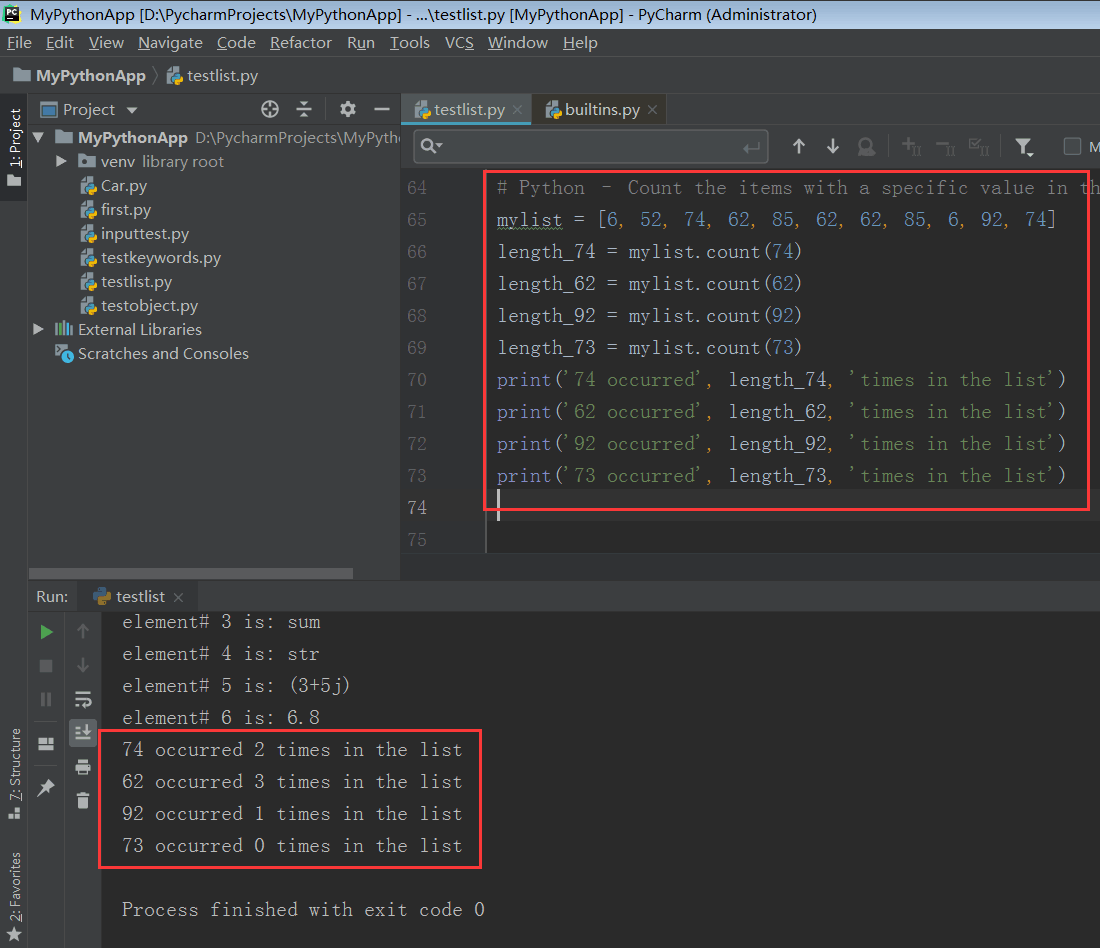count() 函数只需要一个参数。

# 9. 将一张列表追加到另一张列表

list1.extend(list2)


## 9.1. 追加列表到另一个列表

# Append a list to another list
list1 = [6, 52, 74, 62]
list2 = [85, 17, 81, 92]
list1.extend(list2)
print(list1)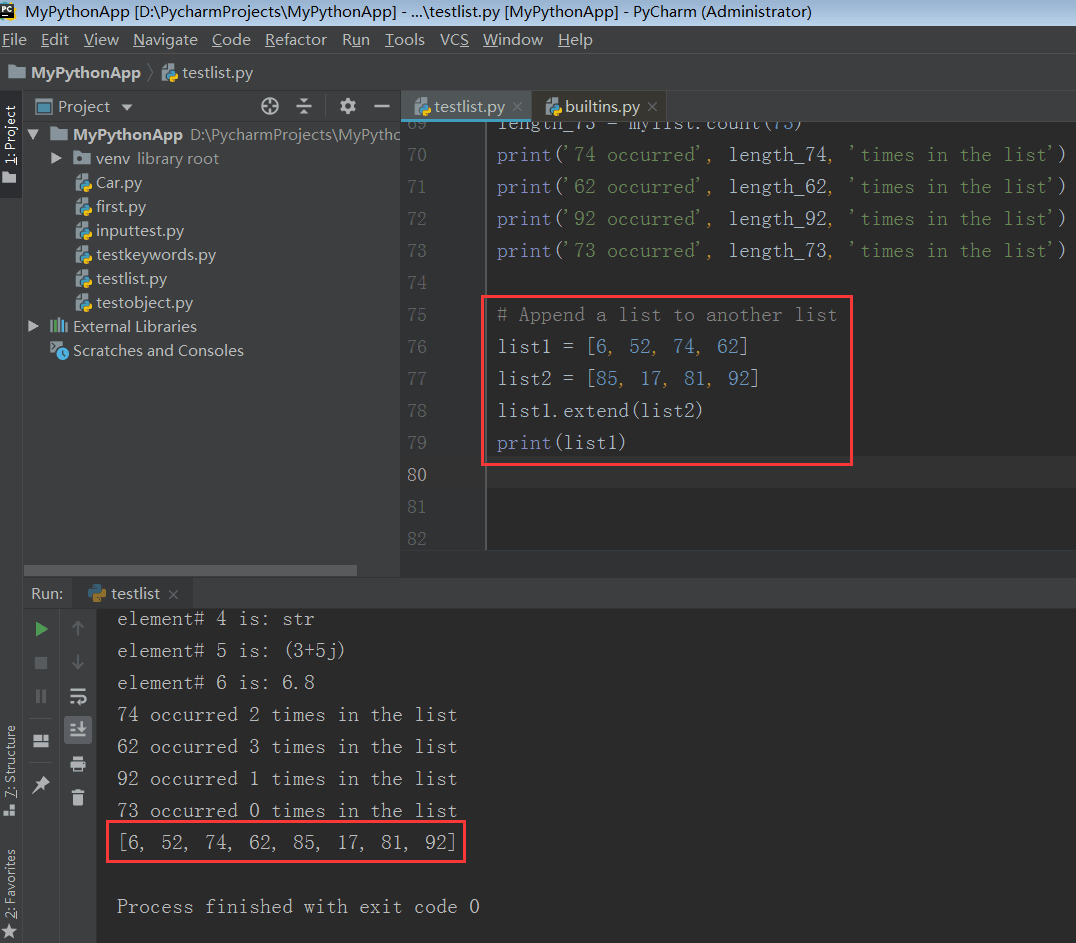list1 的内容已被修改，list2 的内容被追加到原先元素的后边。## 9.2. 保留原来列表，追加另一张列表

# Append a list to another list keeping a copy of original list
list1 = [6, 52, 74, 62]
list2 = [85, 17, 81, 92]
list = list1.copy()
list.extend(list2)
print(list)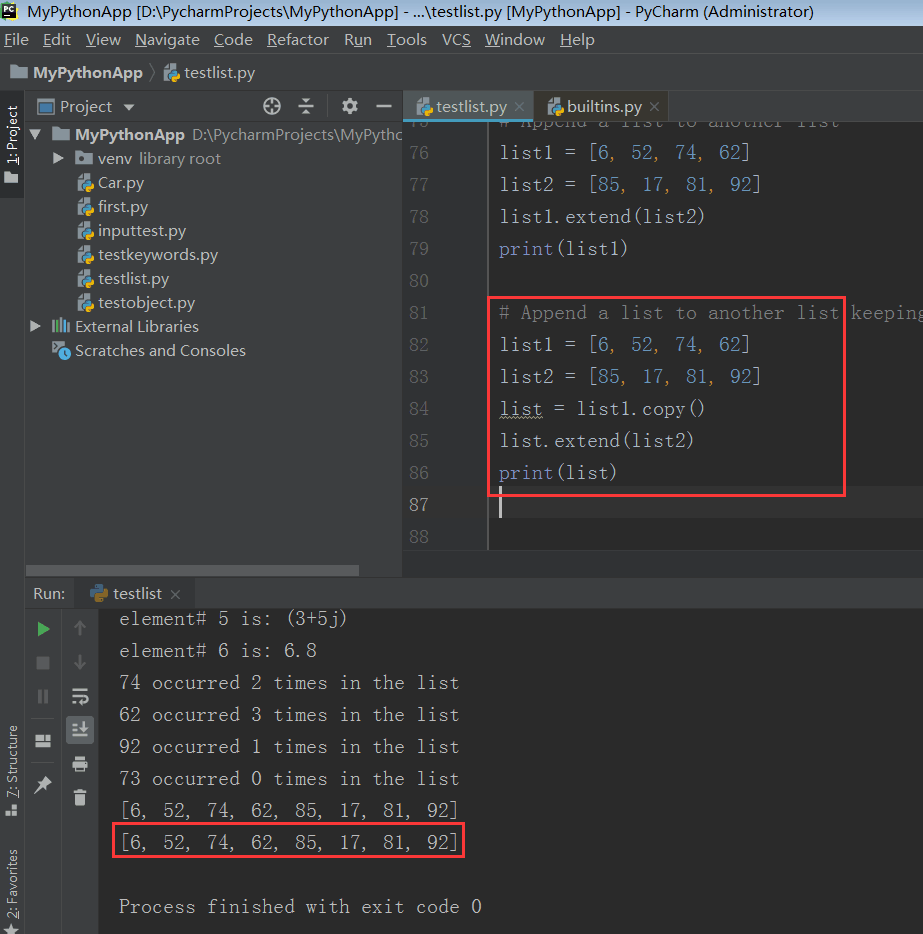# 10. 查找元素在列表中的索引

index = mylist.index(item)


## 10.1. 查找列表中某项的索引

# Find index when item is present in List
mylist = [21, 5, 8, 52, 21, 87]
item = 8
# search for the item
index = mylist.index(item)
print('The index of', item, 'in the list is:', index)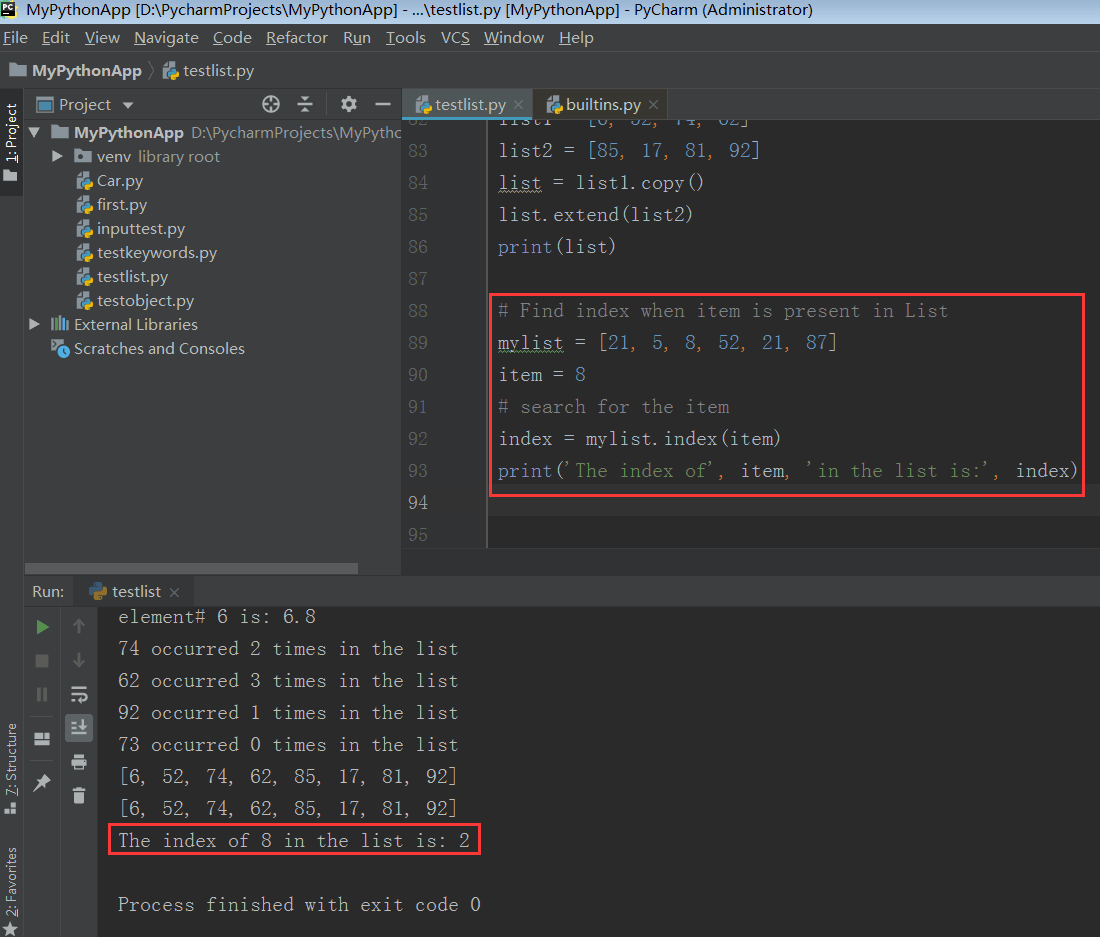## 10.2. 查找列表中某多次出现项的索引

Python 的列表允许同一元素多次出现。这种情况下，只有第一次出现位置的索引会被返回。

# Find index when item is present multiple times in List
mylist = [21, 5, 8, 52, 21, 87, 52]
item = 52
# search for the item
index = mylist.index(item)
print('The index of', item, 'in the list is', index)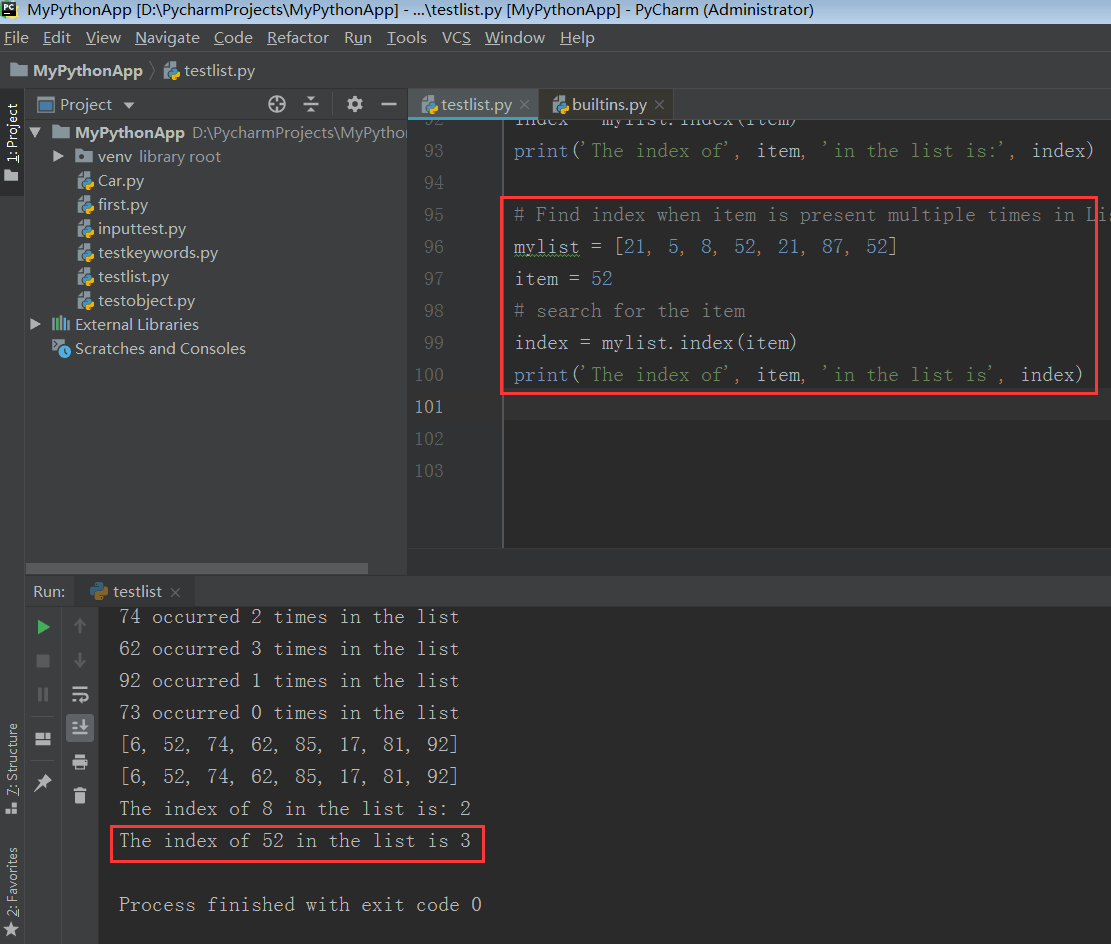## 10.3. 查找没有出现在列表中的项的索引

# Find Index when the specided Item is not present in List
mylist = [21, 5, 8, 52, 21, 87, 52]
item = 67
# search for the item
index = mylist.index(item)
print('The index of', item, 'in the list is', index)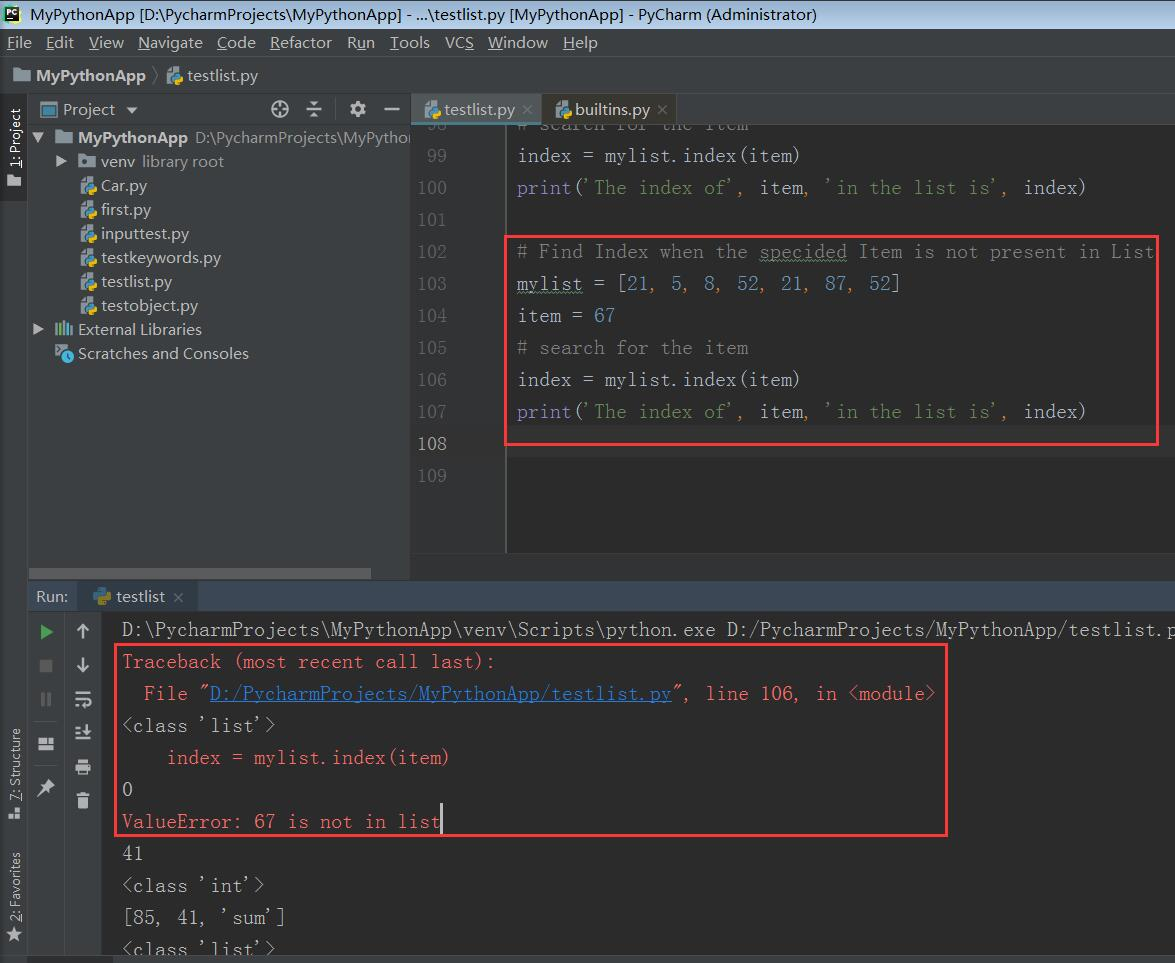# 11. 在指定索引处插入元素

mylist.insert(index, item)


## 11.1. 在指定索引处插入元素

# Insert Item at Specified Index in List
mylist = [21, 5, 8, 52, 21, 87, 52]
item = 36
index = 4
# insert item in mylist at index
mylist.insert(index, item)
print(mylist)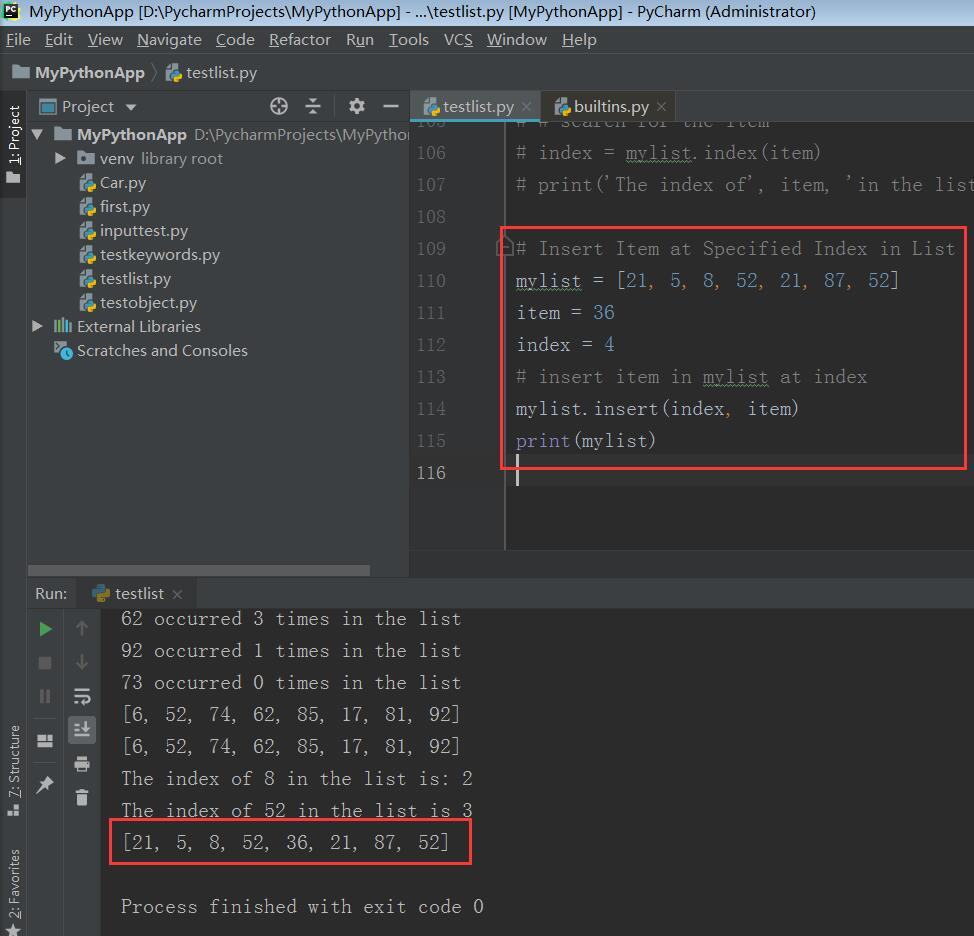## 11.2. 在列表头插入元素

# Insert Item at Start of List
mylist = [21, 5, 8, 52, 21, 87, 52]
item = 36
index = 0 # 1st position
# insert item in mylist at index
mylist.insert(index, item)
print(mylist)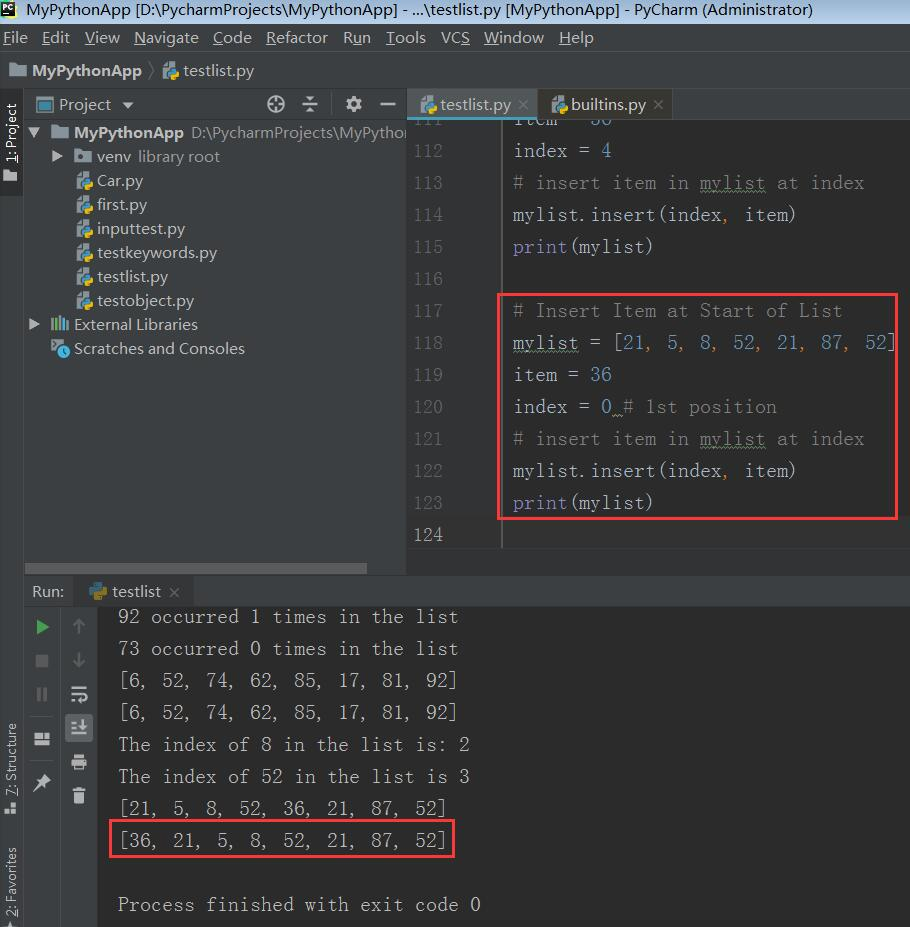## 11.3. 在列表尾插入元素

# Insert Item at End of List
mylist = [21, 5, 8, 52, 21, 87, 52]
item = 36
index = len(mylist)
# insert item in mylist at index
mylist.insert(index, item)
print(mylist)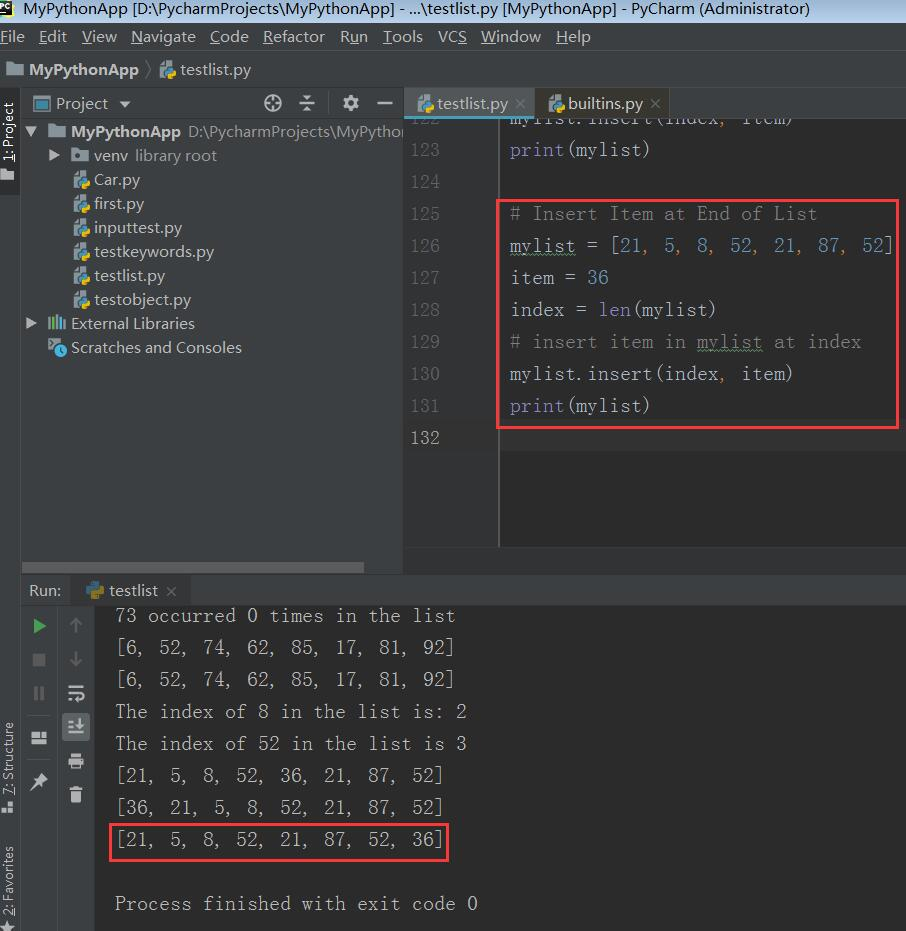## 11.4. 插入数组越界的元素

# Insert Item with Index out of Bounds of List
mylist = [21, 5, 8, 52, 21, 87, 52]
item = 36
index = 1000 # index out of bounds of list
# insert item in mylist at index
mylist.insert(index, item)
print(mylist)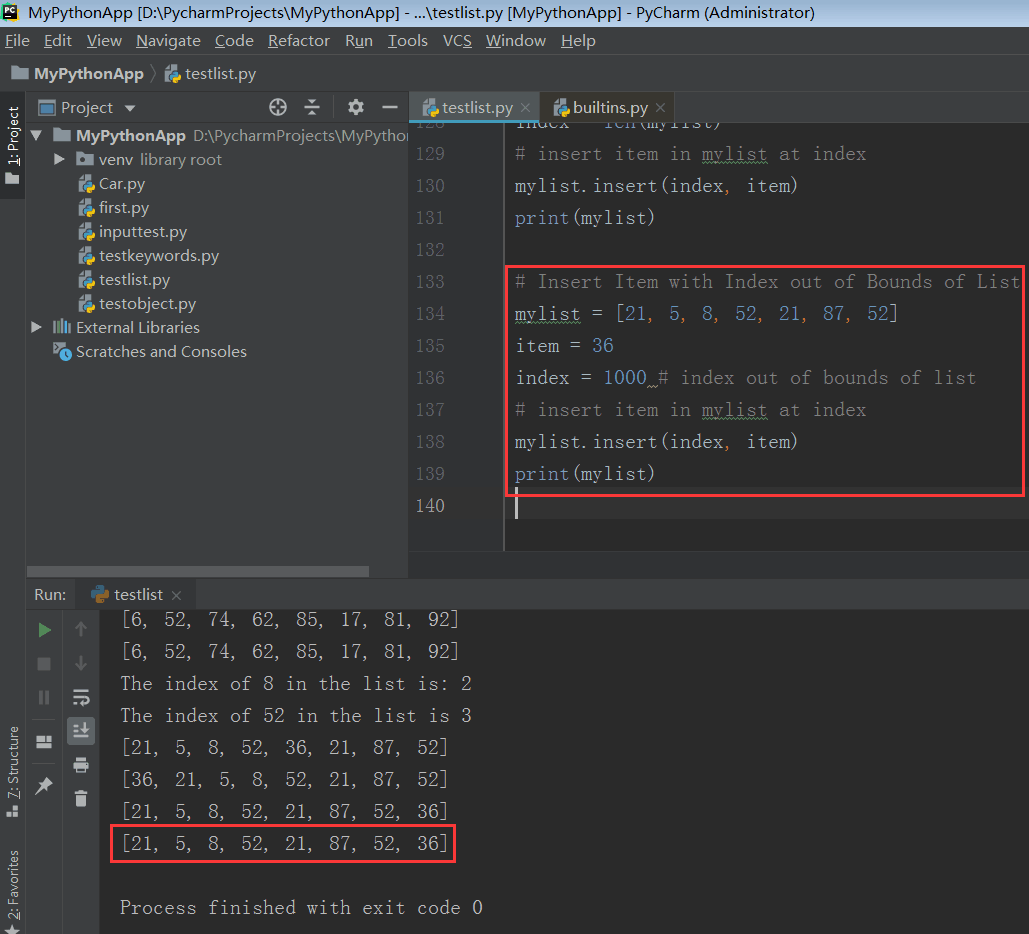mylist = [21, 5, 8, 52, 21, 87, 52]
item = 36
index = -10 # index out of bounds of list
# insert item in mylist at index
mylist.insert(index, item)
print(mylist)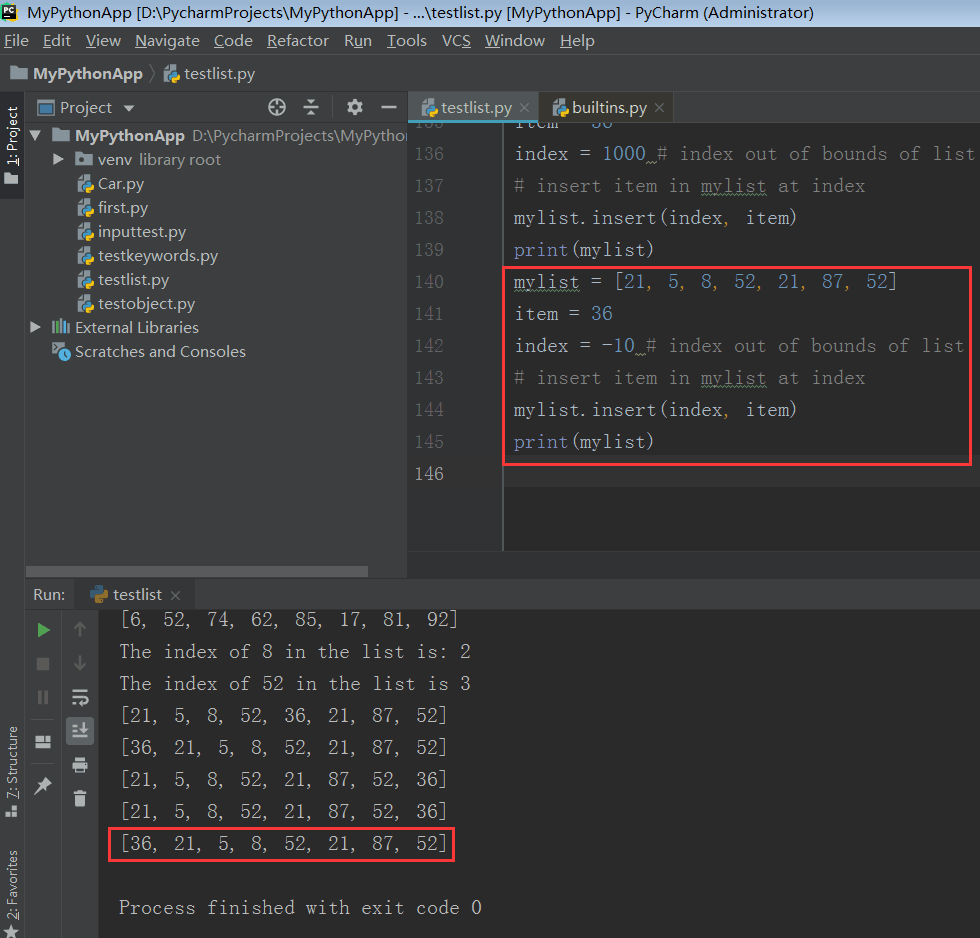# 12. 在指定索引处移除元素

mylist.pop(index)


## 12.1. 在指定索引处移除元素

# Remove Item at Specific Index from List
mylist = [21, 5, 8, 52, 21, 87, 52]
index = 3
# delete item in mylist at index
mylist.pop(index)
print(mylist)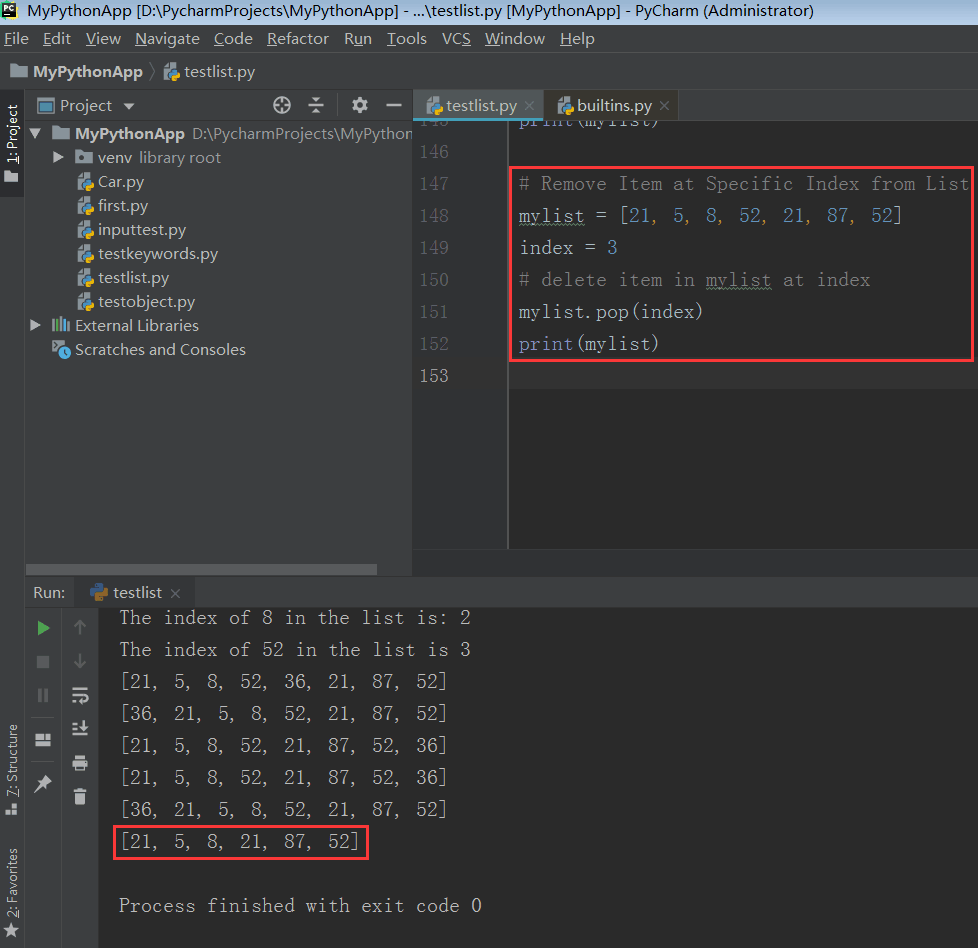## 12.2. 移除列表的最后一个元素

# Remove Last Item of List
mylist = [21, 5, 8, 52, 21, 87, 52]
# delete last item in mylist
mylist.pop()
print(mylist)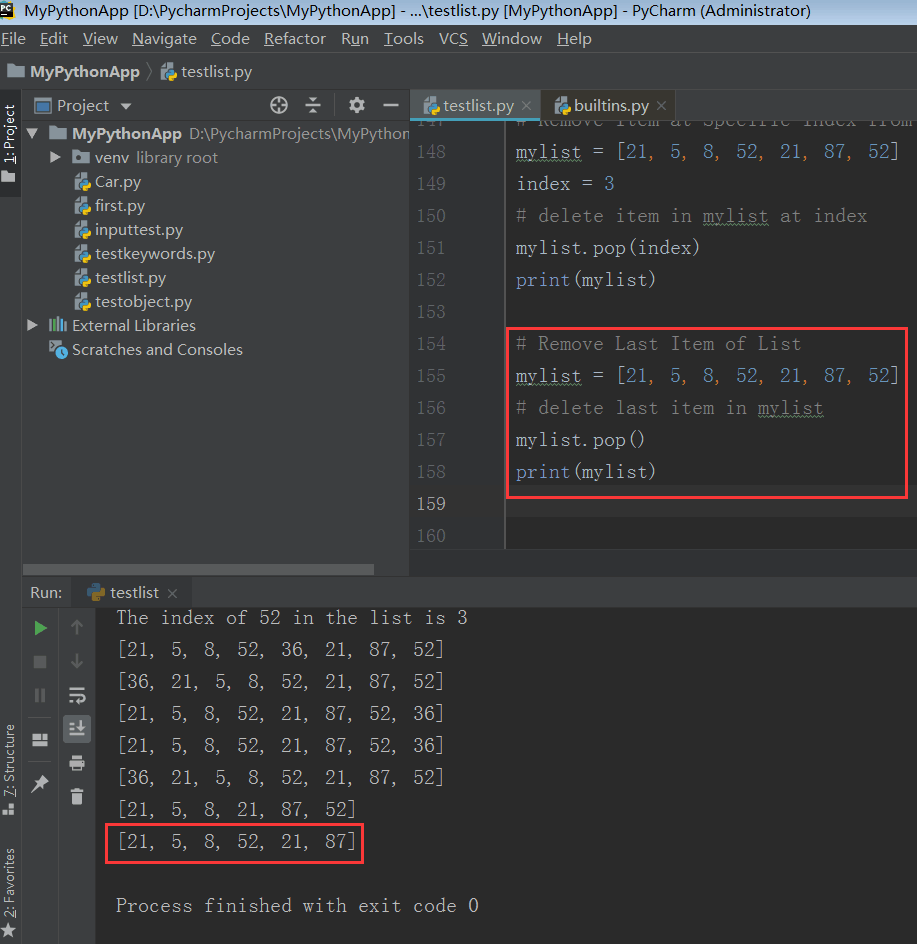## 12.3. 将超出列表长度的索引传递给 pop() 方法

# pop() method with index > length of list
mylist = [21, 5, 8, 52, 21, 87, 52]
index = 100 # index > length of list
mylist.pop(index)
print(mylist)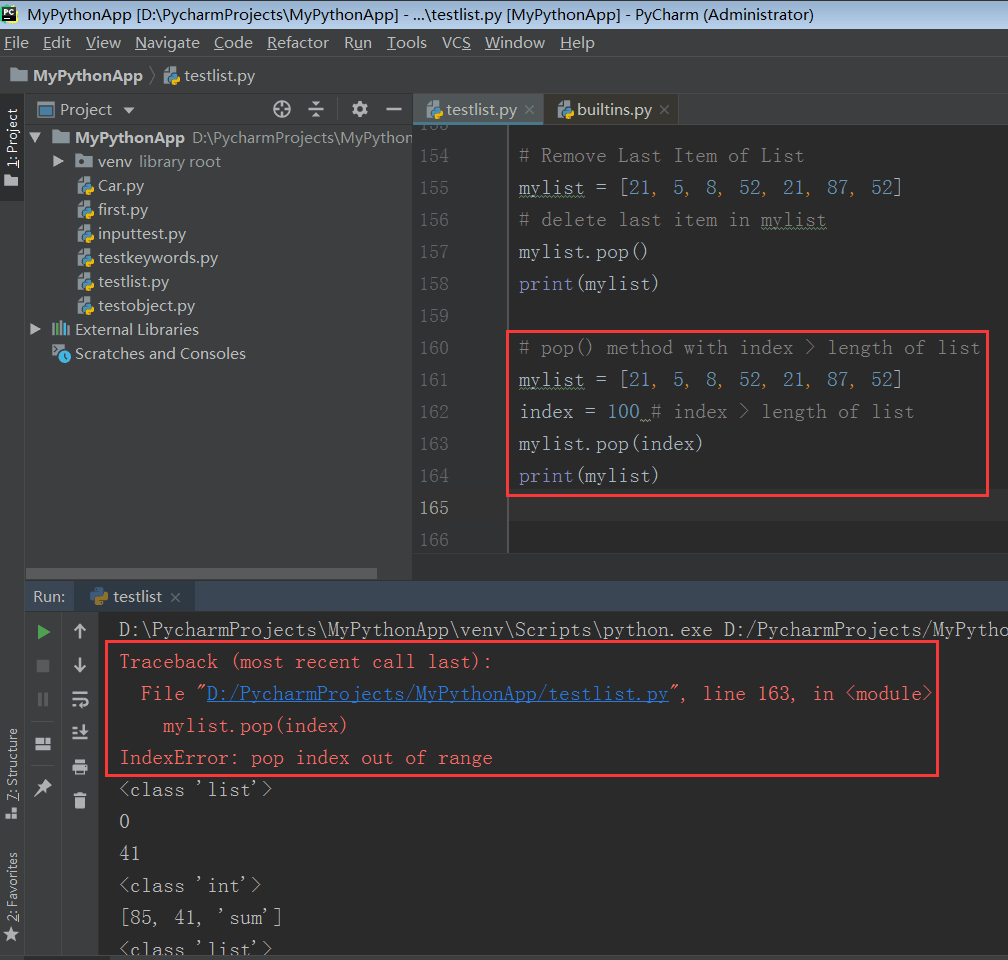## 12.4. 将负值索引传递给 pop() 方法

# pop() with negative index
mylist = [21, 5, 8, 52, 21, 87, 52]
index = -2 # index < 0
mylist.pop(index)
print(mylist)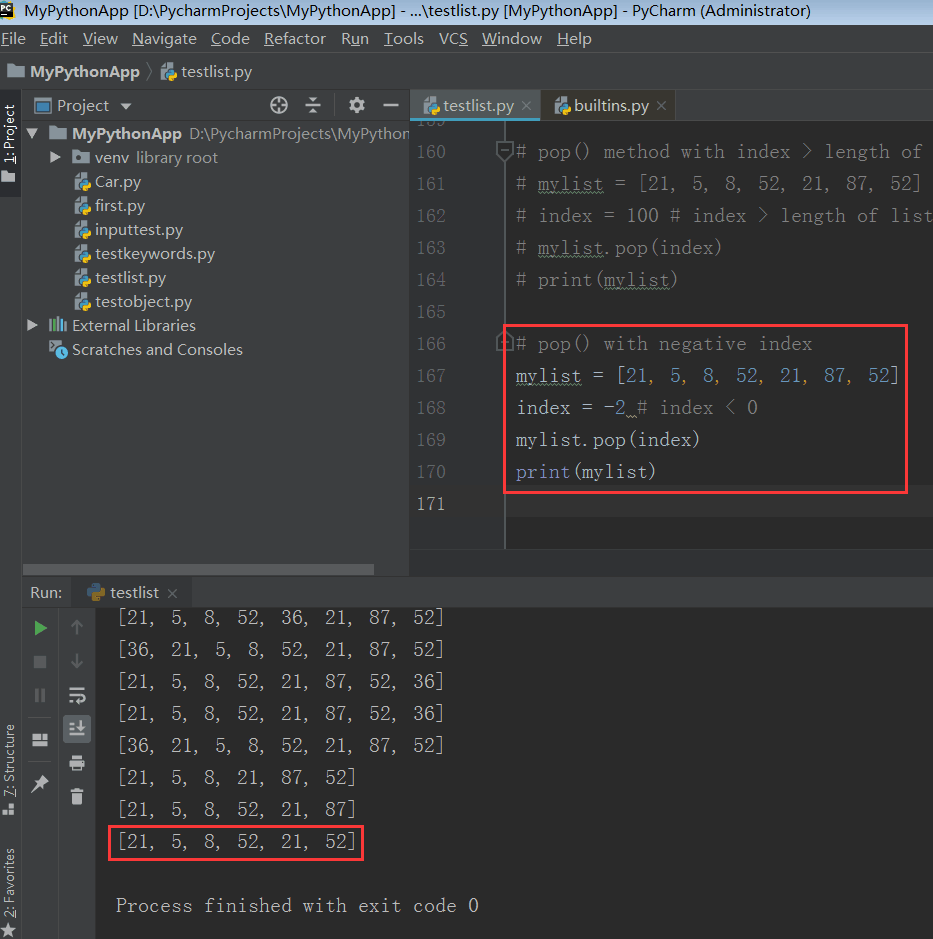# 13. 列表反转

mylist.reverse()

reversed_list = mylist[::-1]

## 13.1. 使用 reverse() 反转列表

# Reverse List using reverse()
mylist = [21, 5, 8, 52, 21, 87, 52]
mylist.reverse()
print(mylist)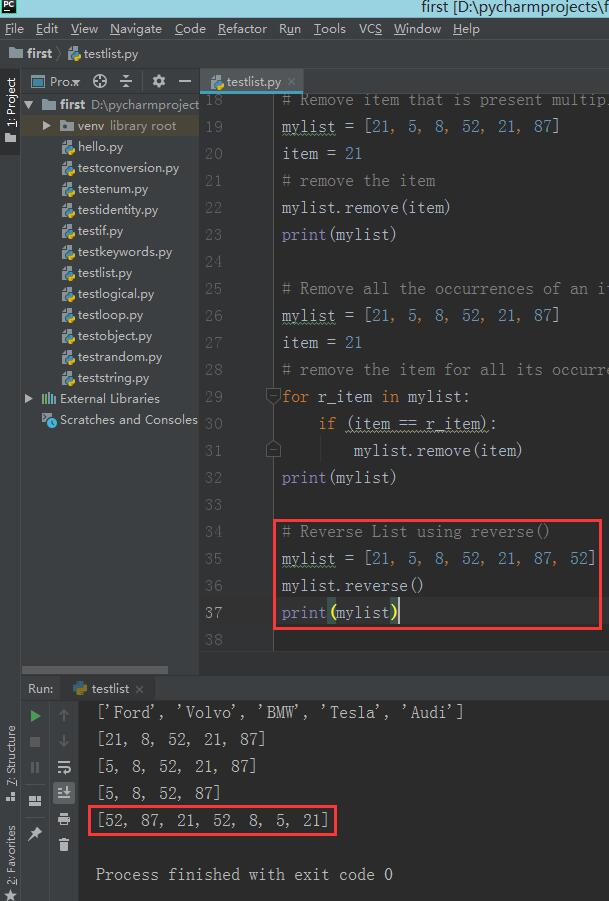## 13.2. 使用切片反转列表

# Reverse List using Slicing
mylist = [21, 5, 8, 52, 21, 87, 52]
mylist2 = mylist[ : : -1]
print(mylist)
print(mylist2)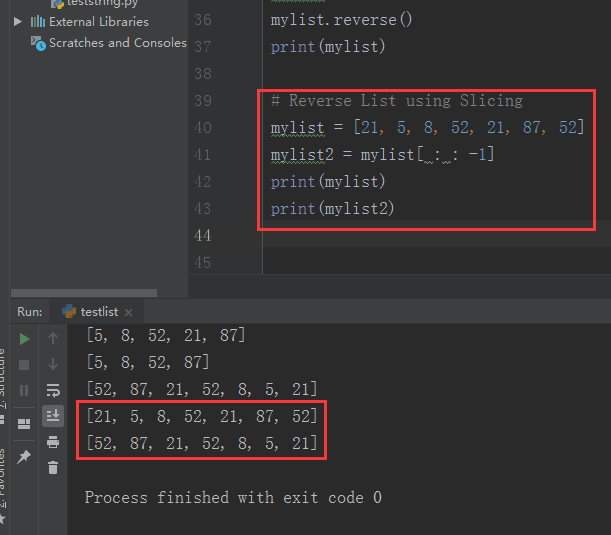## 13.3. 反转字符串列表

# Reverse List of Strings
mylist = ['list', 'dict', 'set']
mylist.reverse()
print(mylist)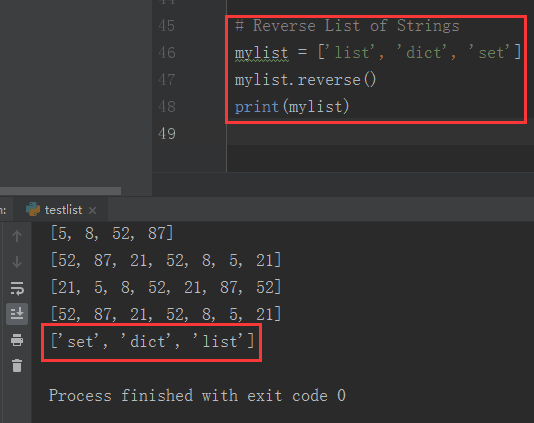## 13.4. 列表反转的应用

• 通过反转列表，你改变了列表中元素的存储次序。如果列表中元素原来已经正序排列，那么通过列表反转，你将得到一个逆序排列。

# 14. 列表排序

mylist.sort(cmp=None, key=None, reverse=False)


• cmp 定义了一个自定义的比较函数，它需要两个参数，返回一个负数，零或正数，这取决于第一个参数比第二个参数小，相等或大。比如：cmp=lambda x,y: cmp(x.lower(), y.lower())
• key 定义了一个函数，它有一个参数，用于从每个列表元素中提取比较键：key=str.lower。默认值为 None (即直接对元素进行比较)。
• reverse 是一个布尔值。如果设置为 True，列表元素将会按照比较结果反转排列。

## 14.1. 对列表正序排序

# Sort a List in Ascending Order
mylist = [21, 5, 8, 52, 21, 87, 52]
mylist.sort()
print(mylist)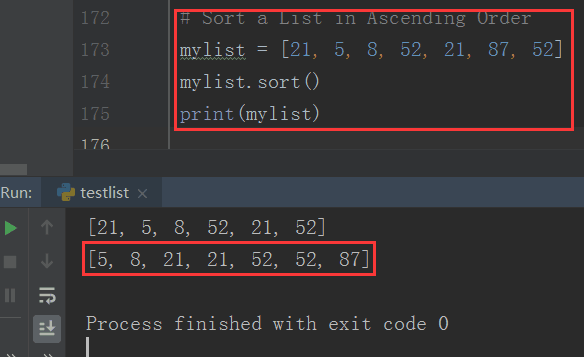sort() 函数的默认行为是对列表元素进行正序排序。

## 14.2. 对列表逆序排序

# Sort a List in Descending Order
mylist = [21, 5, 8, 52, 21, 87, 52]
mylist.sort(reverse=True)
print(mylist)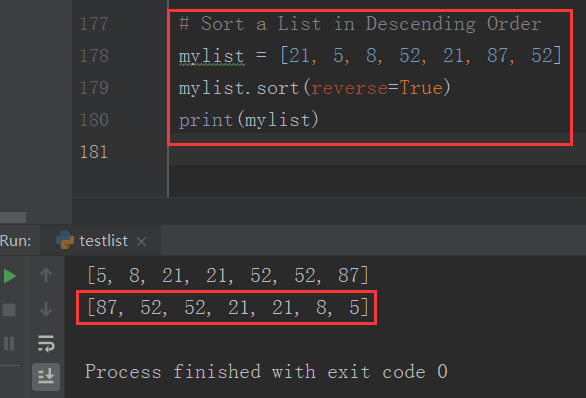# 15. 找到一张列表中最大的数字

## 15.1. 使用 sort() 函数找到最大数字

# Find the largest number using sort() function
a = [18, 52, 23, 41, 32]
a.sort()
a_len = len(a)
ln = a[a_len - 1]
print('Largest element is', ln)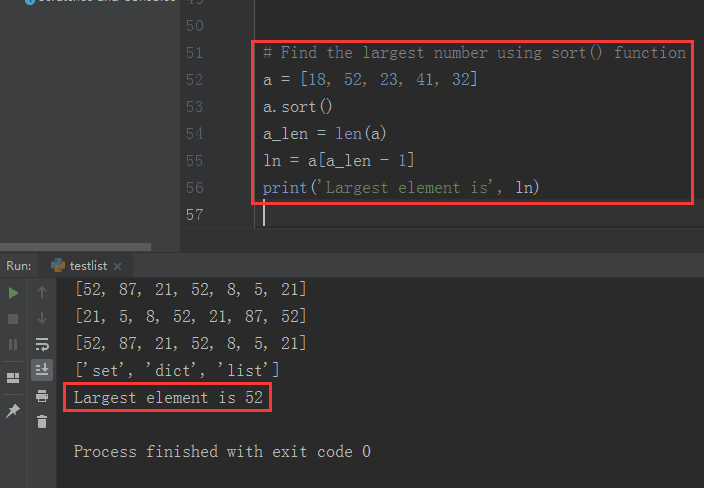## 15.2. 使用 for 循环找到最大数字

# Find the largest number using for loop
a = [18, 52, 23, 41, 32]
ln = 0
for i in a:
if i > ln:
ln = i
print('Largest element is', ln)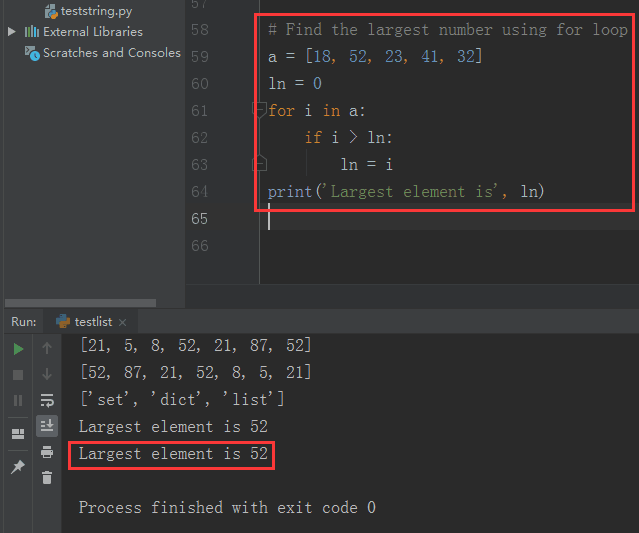# 16. 找到一张列表中最小的数字

## 16.1. 使用 sort() 函数找到最小数字

# Find the smallest number using sort() function
a = [18, 52, 23, 41, 32]
a.sort()
sn = a
print('Smallest element is', sn)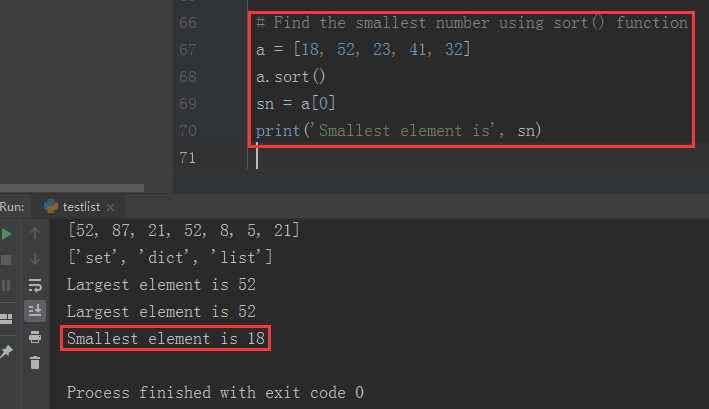## 16.2. 使用 for 循环找到最小数字

# Find the smallest number using for loop
a = [18, 52, 23, 41, 32]
sn = float("inf")
for i in a:
if i < sn:
sn = i
print('Smallest element is', sn)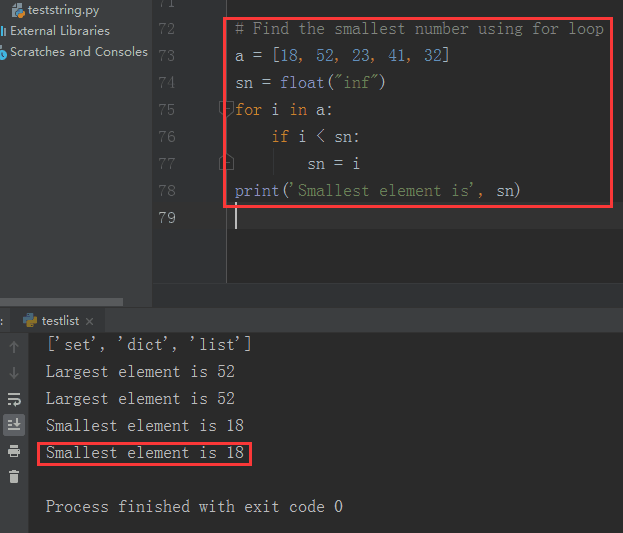# 参考资料

08-0620408-221524
09-02912
04-27278
05-19268
11-09345
04-12194
09-11683
09-011278
04-09578